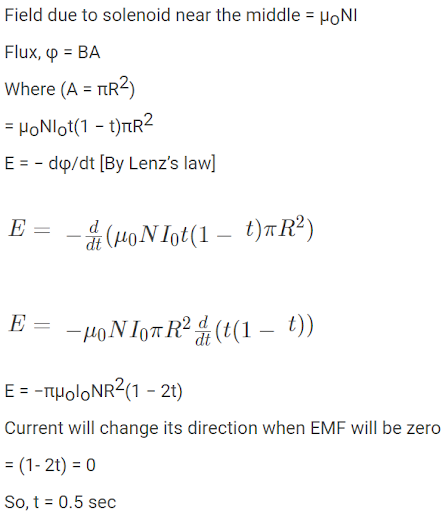# A long solenoid of radius R carries a time (t) dependent current I(t) = I0t(1 − t). A ring of radius 2R is placed coaxially near its middle.

A long solenoid of radius R carries a time (t) dependent current I(t) = I0t(1 − t). A ring of radius 2R is placed coaxially near its middle. During the time instant 0 ≤ t ≤ 1, the induced current (IR) and the induced EMF (VR) in the ring changes as:

1. a. Direction of IR remains unchanged and VR is maximum at t = 0.5
2. b. Direction of IR remains unchanged and VR is zero at t = 0.25
3. c. At t = 0.5 direction of IR reverses and VR is zero
4. d. At t = 0.25 direction of IR reverses and VR is maximum

Correct answer is option C